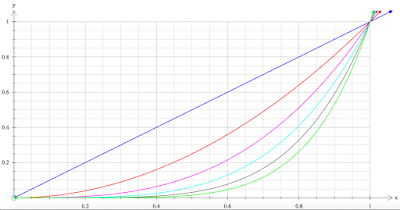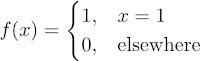Select Page

Firstly, let’s consider what it means to have a sequence of functions by looking closely atAll this is saying is that our sequence isgoing of to infinity. But this is just boring though, what we want to know is what happens when I go to infinity? What does it mean for the sequence of functions to converge? Consider the following picture showing all the terms up to:Graphically, you can see that all the functions are coming closer and closer to being one shape. The functions will always beatand they approacheverywhere else. So from the picture, we can say that our sequence of functionsconverges to:So we can write:More specifically,converges pointwise to. Clearly there is a problem since the limit of a sequence of continuous functions is not continuous.  So what I want to do next is show you why, using the “delta-epsilon” definition of a limit, it is impossible to have a continuous pointwise limit. This isn’t really a proof, but I thought it was pretty cool, so I’m going to show it to you as a fail of a proof.

Fail of a proof:

Just to re-iterate we will try to prove that the limit of a sequence continuous functions is continuous. We start with the sequenceover the intervalwhich has a pointwise limitand we choose a fixed point. We will use the definition of continuity to prove thatis continuous at our general point, and since this is completely general, if our proof works for, then it will work for any point in the intervalthat our function is defined, so we would have proved thatis continuous on the required interval.

Applying the definition of continuity gives: for allthere exists asuch that ifthen it follows that. Let’s deal with with this last expression:Here we haven’t changed anything, all we have done is added zero twice, that is, the red and blue bits. Then we used the triangle inequality to get the second line. Neat trick!

Now the question is, how do we make each of terms in that second line small,small to be precise, in order to satisfy our definition above. You may be wandering why, and the answer to that is just so we can sum them in the green line and get a total ofwhich matches what we previously stated,. Well clearly, asapproaches, the first and last term go to zero, and, since we knowis continuous, we can use that. So now let’s chooselarge enough such that:Now that we have that and we know thatis continuous, we apply the definition of continuity toat the point. Namely, for allthere exists asuch that ifthen it follows that. So we have successfully, made the middle term of our green line small!

Let’s try to make the first and last termssmall. For this to happen, from the definition of a limit, we need:for allWell here is the problem, and it isn’t quite so clear.

The sameis in both purple bits, however, the samemay not be sufficient for both purple bits.

Why can’t we just choose a bigger? Well the value ofdepends on, because we choose anythat satisfies, and in turn, this value ofdepends on our value of. Why does it depend on N? Well you can imagine higher and highervalues forwill get closer and closer to the limit function. Hencecan become smaller asgets larger (and still satisfy our definition above) and thus the range from which we pickto be is made smaller as well.

Thus we cannot just changewithout changing whichvalues we are talking about, then we wouldn’t really be limiting the our green line properly, just each term randomly.

Evident from the above discussion is thatcan be made true for large enough values of, but this value ofdepends on which x-value we are talking about. To illustrate this consider our example above ofusing. To achieve the inequalitywe just need to go to. However if we change thevalue to, it turns out we need to go toto make sureis true.

So in conclusion, pointwise convergence is crappy! We need a stronger notion of convergence when it comes to sequences and series of functions, absolute convergence, which I might talk about later!